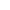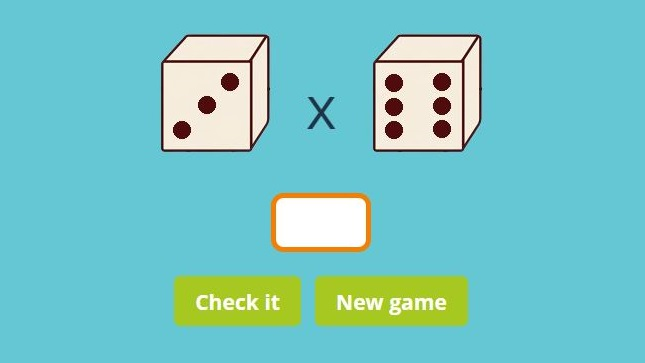﻿ Free Multiplication Dice Games ONLINE + Printable

# Multiplication Dice Games Online

Free dice multiplication games. Free multiplication dice games online 4th grade are a fun way how kids can learn multiplication. Free math dice games for kids. You can also download free printable multiplication dice game worksheet or multiplication dice game in pdf. Play free dice games online free. Play online dice game. Free dice game online multiplayer. dice games multiplication. multiplication dice game printable. Online dice games FREE. multiplication games with dice. educational dice games free online.xNew gameMultiplication dice games online: insert the result to the empty box.

## Free multiplication dice games online freeIf you don't know how to teach children basic multiplication, play with them our free multiplication dice games online. Insert the result of the multiplication dice game to the empty box. Press ´Check it´ to find out the correct answer. If the result is OK, the box will be green.

If your answer is wrong, the box will be red and you will see the correct number. Press the button "New game" and play another game.

## Multiplication dice game in PDF

Click the picture bellow and download our free printable multiplication dice game in PDF with instuctions. You can easily print these free dice multiplication games on A4 or A3 paper. You can browse more printable math games here.

## Dice multiplication games

Click the picture bellow and download our free multiplication dice games in PDF. One dice multiplication worksheet is already pre-filled, the other printable multiplication dice game contains only free fields. This blank dice multiplication game features a lovely sheet with different coloured squares.

How to play multiplication dice game?
You will need a dice, for example this set of 10. Roll the dice twice, insert the numbers in the first two boxes. Multiply these numbers and write the result in the last box.

Browse more multiplication worksheets here.

## Fun multiplication games with a dice

These multiplication games with a dice teach multiplication of numbers 1 to 6. Our multiplication dice game is designed for children who attend 3rd Grade. You can play these multiplication dice games online.

If you’re not interested in online dice games, here are my printable multiplication games. You can easily and quickly print these multiplication games as per your requirements.

Do you want to play more difficult multiplication games? Then try this single digit multiplication game or this multiplication pyramid game.### Multiplication dice game online

Mastering multiplication is one of the most important and difficult tasks in mathematics.

To help our kids to learn one digit multiplication easily we developed these dice multiplication games.

You can also learn about the basic concept of multiplication with the help of little fishes in this video.

## Free online dice games for kids

One of the best ways to keep kids engaged in math lesson is by playing a game! Try out this dice multiplication game to help them practice multiplication while still taking a little breather from other activities.If you don't have a set of dice handy to enjoy our free multiplication games with dice, you can let roll our online dice. Just a few minutes a day can help to cement key concepts of multiplication.

Category: